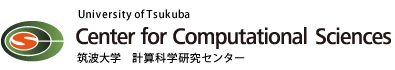### CCSQCDSolverBench

* CCS QCD Solver benchmark program *

[VERSION]

VERSION 0.999 : September 16, 2017 : sequential initialization added to speed up on large volume.
VERSION 0.990 : December 24, 2012 (Fortran Version)

[DESCRIPTION]

This benchmark test program measures CPU and MPI performances.

This program benchmarks the performance of a linear equation solver
with a large sparse coefficient matrix appering in a lattice QCD probrem.
Lattice QCD describes the property of protons, neutrons and neucleons
in terms of more fundamental interacting elementary particles gluon and
quarks.

The four dimensional (spase and time) coordinate is latticized and the
equation of motion for quarks is converted to a large scale linear equation
by the finite-difference method.

The coefficient matrix of the induced linear equation used in this program
is the O(a)-improved Wilson-Dirac operator (called Clover fermion).
This benchmark program measures the performance of solving the follwing
linear equation:

D x = b,

where D is the clover fermion coefficient matrix defined on full lattice.
b is a source spinor-field, and x is the unknown spinor-field to be solved.
The linear equation is preconditioned by the site red-brack ordering and
the preconditioned equation is solved with BiCGStab algorithm.

See “README” file for the details.

[CONTACT]

CCS QCD solver benchmark project

Ken-Ichi Ishikawa (Hiroshima University)
Yoshinobu Kuramashi (University of Tsukuba)
Akira Ukawa (University of Tsukuba)
Taisuke Boku (University of Tsukuba)

Center for Computational Sciences
University of Tsukuba
1-1-1 Tennodai
Tsukuba-city
Ibaraki 305-8577
Japan

URL: http://www.ccs.tsukuba.ac.jp/
EMAIL: ccsqcdbench[_AT_]ccs.tsukuba.ac.jp